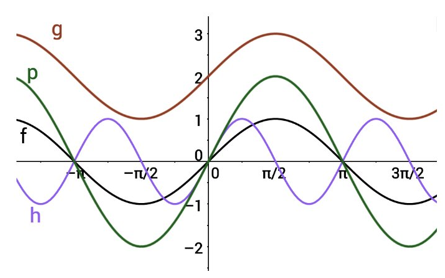# Unit Circle and Trigonometric Functions

## Objective

Graph transformations of sine and cosine functions (Part I).

## Common Core Standards

### Core Standards

?

• F.IF.C.7.E — Graph exponential and logarithmic functions, showing intercepts and end behavior, and trigonometric functions, showing period, midline, and amplitude.

• F.TF.A.1 — Understand radian measure of an angle as the length of the arc on the unit circle subtended by the angle.

• F.TF.A.3 — Use special triangles to determine geometrically the values of sine, cosine, tangent for π/3, π/4 and π/6, and use the unit circle to express the values of sine, cosine, and tangent for π-x, π+x, and 2π-x in terms of their values for x, where x is any real number.

• F.TF.A.4 — Use the unit circle to explain symmetry (odd and even) and periodicity of trigonometric functions.

## Criteria for Success

?

1. Describe how the graphs of sine and cosine functions relate to the unit circle.
2. Identify amplitude, period, and midline of sine and cosine functions.
3. Identify maximum and minimum values of sine and cosine functions.
4. Explain why the sine function is odd and the cosine function is even.

## Tips for Teachers

?

• This GeoGebra tool, Unit Circle and Sine Graph, draws the graph from the unit circle.
• Use radians for any materials from here on out even though the referenced Algebra 2 EngageNY lesson has the material in degrees.
• Focus on getting students practice with simple transformations of sine and cosine functions.

## Anchor Problems

?

### Problem 1

{}<{}p{}>{}T{}h{}e{} {}p{}o{}i{}n{}t{}s{} {}o{}n{} {}t{}h{}e{} {}g{}r{}{{}a{}}{}p{}h{}s{} {}{{}a{}}{}n{}{{}d{}}{} {}t{}h{}e{} {}u{}n{}i{}t{} {}{{}c{}}{}i{}r{}{{}c{}}{}l{}e{} {}{{}b{}}{}e{}l{}o{}w{} {}w{}e{}r{}e{} {}{{}c{}}{}h{}o{}s{}e{}n{} {}s{}o{} {}t{}h{}{{}a{}}{}t{} {}t{}h{}e{}r{}e{} {}i{}s{} {}{{}a{}}{} {}r{}e{}l{}{{}a{}}{}t{}i{}o{}n{}s{}h{}i{}p{} {}{{}b{}}{}e{}t{}w{}e{}e{}n{} {}t{}h{}e{}m{}.{} {}E{}x{}p{}l{}{{}a{}}{}i{}n{} {}t{}h{}e{} {}r{}e{}l{}{{}a{}}{}t{}i{}o{}n{}s{}h{}i{}p{} {}{{}b{}}{}e{}t{}w{}e{}e{}n{} {}t{}h{}e{} {}{{}c{}}{}o{}o{}r{}{{}d{}}{}i{}n{}{{}a{}}{}t{}e{}s{}&{}n{}{{}b{}}{}s{}p{};{}<{}s{}p{}{{}a{}}{}n{} {}{{}c{}}{}l{}{{}a{}}{}s{}s{}={}"{}m{}{{}a{}}{}t{}h{}-{}t{}e{}x{}"{}>{}${}${}{{}a{}}{}${}${}<{}/{}s{}p{}{{}a{}}{}n{}>{},{}&{}n{}{{}b{}}{}s{}p{};{}<{}s{}p{}{{}a{}}{}n{} {}{{}c{}}{}l{}{{}a{}}{}s{}s{}={}"{}m{}{{}a{}}{}t{}h{}-{}t{}e{}x{}"{}>{}${}${}{{}b{}}{}${}${}<{}/{}s{}p{}{{}a{}}{}n{}>{},{}&{}n{}{{}b{}}{}s{}p{};{}<{}s{}p{}{{}a{}}{}n{} {}{{}c{}}{}l{}{{}a{}}{}s{}s{}={}"{}m{}{{}a{}}{}t{}h{}-{}t{}e{}x{}"{}>{}${}${}{{}c{}}{}${}${}<{}/{}s{}p{}{{}a{}}{}n{}>{},{} {}{{}a{}}{}n{}{{}d{}}{}&{}n{}{{}b{}}{}s{}p{};{}<{}s{}p{}{{}a{}}{}n{} {}{{}c{}}{}l{}{{}a{}}{}s{}s{}={}"{}m{}{{}a{}}{}t{}h{}-{}t{}e{}x{}"{}>{}${}${}{{}d{}}{}${}${}<{}/{}s{}p{}{{}a{}}{}n{}>{}&{}n{}{{}b{}}{}s{}p{};{}m{}{{}a{}}{}r{}k{}e{}{{}d{}}{} {}o{}n{} {}t{}h{}e{} {}g{}r{}{{}a{}}{}p{}h{} {}o{}f{}&{}n{}{{}b{}}{}s{}p{};{}<{}s{}p{}{{}a{}}{}n{} {}{{}c{}}{}l{}{{}a{}}{}s{}s{}={}"{}m{}{{}a{}}{}t{}h{}-{}t{}e{}x{}"{}>{}${}${}y{}={}\{}m{}{{}a{}}{}t{}h{}r{}m{}{{}s{}i{}n{}}{}t{}${}${}<{}/{}s{}p{}{{}a{}}{}n{}>{}&{}n{}{{}b{}}{}s{}p{};{}{{}a{}}{}n{}{{}d{}}{}&{}n{}{{}b{}}{}s{}p{};{}<{}s{}p{}{{}a{}}{}n{} {}{{}c{}}{}l{}{{}a{}}{}s{}s{}={}"{}m{}{{}a{}}{}t{}h{}-{}t{}e{}x{}"{}>{}${}${}y{}={}\{}m{}{{}a{}}{}t{}h{}r{}m{}{{}{{}c{}}{}o{}s{}}{}t{}${}${}<{}/{}s{}p{}{{}a{}}{}n{}>{}&{}n{}{{}b{}}{}s{}p{};{}{{}a{}}{}n{}{{}d{}}{} {}t{}h{}e{} {}q{}u{}{{}a{}}{}n{}t{}i{}t{}i{}e{}s{}&{}n{}{{}b{}}{}s{}p{};{}<{}s{}p{}{{}a{}}{}n{} {}{{}c{}}{}l{}{{}a{}}{}s{}s{}={}"{}m{}{{}a{}}{}t{}h{}-{}t{}e{}x{}"{}>{}${}${}{{}A{}}{}${}${}<{}/{}s{}p{}{{}a{}}{}n{}>{},{}&{}n{}{{}b{}}{}s{}p{};{}<{}s{}p{}{{}a{}}{}n{} {}{{}c{}}{}l{}{{}a{}}{}s{}s{}={}"{}m{}{{}a{}}{}t{}h{}-{}t{}e{}x{}"{}>{}${}${}{{}B{}}{}${}${}<{}/{}s{}p{}{{}a{}}{}n{}>{},{}&{}n{}{{}b{}}{}s{}p{};{}{{}a{}}{}n{}{{}d{}}{}&{}n{}{{}b{}}{}s{}p{};{}<{}s{}p{}{{}a{}}{}n{} {}{{}c{}}{}l{}{{}a{}}{}s{}s{}={}"{}m{}{{}a{}}{}t{}h{}-{}t{}e{}x{}"{}>{}${}${}{{}C{}}{}${}${}<{}/{}s{}p{}{{}a{}}{}n{}>{}&{}n{}{{}b{}}{}s{}p{};{}m{}{{}a{}}{}r{}k{}e{}{{}d{}}{} {}i{}n{} {}t{}h{}e{} {}{{}d{}}{}i{}{{}a{}}{}g{}r{}{{}a{}}{}m{} {}o{}f{} {}t{}h{}e{} {}u{}n{}i{}t{} {}{{}c{}}{}i{}r{}{{}c{}}{}l{}e{} {}{{}b{}}{}e{}l{}o{}w{}.{}<{}/{}p{}>{} {} {} {} {}<{}p{} {}s{}t{}y{}l{}e{}={}"{}t{}e{}x{}t{}-{}{{}a{}}{}l{}i{}g{}n{}:{}{{}c{}}{}e{}n{}t{}e{}r{}"{}>{}<{}i{}m{}g{} {}{{}a{}}{}l{}t{}={}"{}"{} {}s{}r{}{{}c{}}{}={}"{}h{}t{}t{}p{}s{}:{}/{}/{}{{}d{}}{}3{}7{}s{}u{}8{}u{}1{}z{}9{}y{}n{}i{}e{}.{}{{}c{}}{}l{}o{}u{}{{}d{}}{}f{}r{}o{}n{}t{}.{}n{}e{}t{}/{}u{}p{}l{}o{}{{}a{}}{}{{}d{}}{}s{}/{}2{}0{}1{}8{}/{}0{}2{}/{}2{}6{}/{}{{}a{}}{}l{}g{}2{}u{}6{}l{}9{}_{}{{}a{}}{}t{}1{}.{}P{}N{}G{}"{} {}s{}t{}y{}l{}e{}={}"{}h{}e{}i{}g{}h{}t{}:{}4{}4{}4{}p{}x{};{} {}w{}i{}{{}d{}}{}t{}h{}:{}3{}0{}0{}p{}x{}"{} {}/{}>{}<{}s{}p{}{{}a{}}{}n{} {}{{}c{}}{}l{}{{}a{}}{}s{}s{}={}"{}m{}{{}a{}}{}t{}h{}-{}t{}e{}x{}"{}>{}${}${}${}${}<{}/{}s{}p{}{{}a{}}{}n{}>{}<{}/{}p{}>{}

#### References

Illustrative Mathematics Trig Functions and the Unit Circle

Trig Functions and the Unit Circle, accessed on Feb. 26, 2018, 3:25 p.m., is licensed by Illustrative Mathematics under either the CC BY 4.0 or CC BY-NC-SA 4.0. For further information, contact Illustrative Mathematics.

### Problem 2

Of sine and cosine, one function is even and the other is odd. Why is this the case?

### Problem 3

Match each graph with its equation.${y=\mathrm{sin}x}$

${y=2\mathrm{sin}x}$

${y=\mathrm{sin}(2x)}$

${y=\mathrm{sin}x}+2$

#### References

New Visions for Public Schools Sine Graphs and Equations

Sine Graphs and Equations is made available by New Visions for Public Schools under the CC BY-NC-SA 4.0 license. © 2017 New Visions for Public Schools. Accessed https://curriculum.newvisions.org/math/resources/resource/algebra-ii-unit-3-big-idea-4-sine-graphs-and-equations/.

Modified by The Match Foundation, Inc.

## Problem Set

?

The following resources include problems and activities aligned to the objective of the lesson that can be used to create your own problem set.

• Mark the coordinate points on the graph for the values in the unit circle.
• Include problems graphing simple transformations of sine and cosine function, as in the third anchor problem.

?

### Problem 1

Sketch a graph of the sine function on the interval ${0<x<2\pi}$ showing all key points of the graph (vertical and horizontal intercepts and maximum and minimum points). Mark the coordinates of the maximum and minimum points and the intercepts.

#### References

EngageNY Mathematics Algebra II > Module 2 > Topic A > Lesson 8Exit Ticket, Question #1

Algebra II > Module 2 > Topic A > Lesson 8 of the New York State Common Core Mathematics Curriculum from EngageNY and Great Minds. © 2015 Great Minds. Licensed by EngageNY of the New York State Education Department under the CC BY-NC-SA 3.0 US license. Accessed Dec. 2, 2016, 5:15 p.m..

Modified by The Match Foundation, Inc.

### Problem 2

Sketch a graph of the cosine function on the interval ${0<x<2\pi}$ showing all key points of the graph (vertical and horizontal intercepts and maximum and minimum points). Mark the coordinates of the maximum and minimum points and the intercepts.

#### References

EngageNY Mathematics Algebra II > Module 2 > Topic A > Lesson 8Exit Ticket, Question #2

Algebra II > Module 2 > Topic A > Lesson 8 of the New York State Common Core Mathematics Curriculum from EngageNY and Great Minds. © 2015 Great Minds. Licensed by EngageNY of the New York State Education Department under the CC BY-NC-SA 3.0 US license. Accessed Dec. 2, 2016, 5:15 p.m..

Modified by The Match Foundation, Inc.